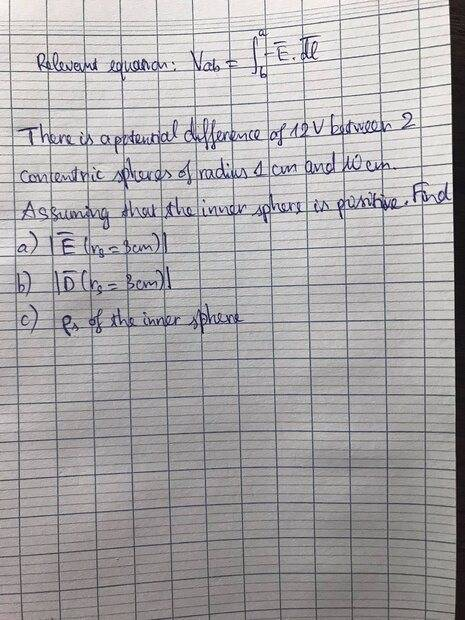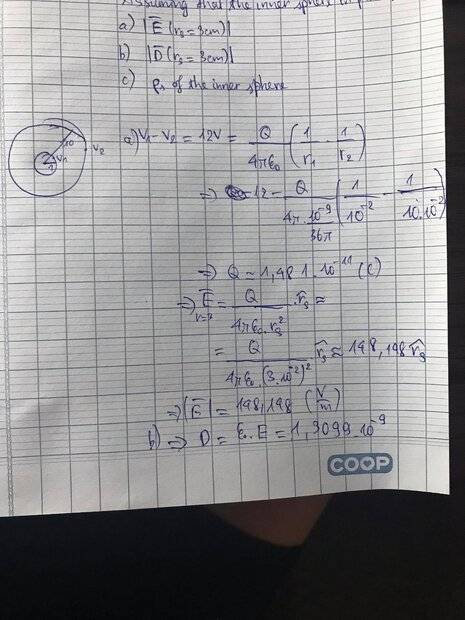# Find electric field intensity/density with given potential difference

vboyn12
Homework Statement:
In photo below. (help me please)
Relevant Equations:
I don't know whether the relevant equation can help because I haven't figure out any idea yet.Homework Helper
Hi,

PF rules (please read them) only allow us to help if you post an attempt at solution.
What have you learned so far, that you might use here?

##\ ##

vboyn12vboyn12
Hi,

PF rules (please read them) only allow us to help if you post an attempt at solution.
What have you learned so far, that you might use here?

##\ ##
Hi, I try to solve part a and b and i posted below, can you have a look at it?

•BvU
Homework Helper
OK, so you use $$V_1-V_2 = 12\ \text{V}\ = {Q\over 4\pi\varepsilon_0}\left ( {1\over r_1} - {1\over r_2} \right )$$(which I suppose might make a good entry in the list of relevant equations)

And then (another one !) $$\left | \vec E(r_s) \right | = {Q\over 4\pi\varepsilon_0}\ {1\over r_s^2}$$so that $$\left | \vec E(r_s) \right | = { 12\ \text{V}\ \over \ \displaystyle {1\over r_1} - {1\over r_2}\ } \ {1\over r_s^2} = 12\ \text{V}\ {1\over r_2-r_1}\;{r_1 r_2\over r_s^2 }$$(thus avoiding calculator and rounding errors!) which agrees with your result once we learn to read your handwritten 4 that looks scaringly similar to a 9I wouldn't quote 6 digits if the ##r## are given in only 1 digit, but that's a matter of taste.
Be sure to use the right ##\varepsilon## if you quote so many digits (I get ##|D| = 1.312 \ 10^{-9} ## !) and do use units !For part c) you need to know that at least the inner sphere is non-conducting !

##\ ##

vboyn12
OK, so you use $$V_1-V_2 = 12\ \text{V}\ = {Q\over 4\pi\varepsilon_0}\left ( {1\over r_1} - {1\over r_2} \right )$$(which I suppose might make a good entry in the list of relevant equations)

And then (another one !) $$\left | \vec E(r_s) \right | = {Q\over 4\pi\varepsilon_0}\ {1\over r_s^2}$$so that $$\left | \vec E(r_s) \right | = { 12\ \text{V}\ \over \ \displaystyle {1\over r_1} - {1\over r_2}\ } \ {1\over r_s^2} = 12\ \text{V}\ {1\over r_2-r_1}\;{r_1 r_2\over r_s^2 }$$(thus avoiding calculator and rounding errors!) which agrees with your result once we learn to read your handwritten 4 that looks scaringly similar to a 9I wouldn't quote 6 digits if the ##r## are given in only 1 digit, but that's a matter of taste.
Be sure to use the right ##\varepsilon## if you quote so many digits (I get ##|D| = 1.312 \ 10^{-9} ## !) and do use units !For part c) you need to know that at least the inner sphere is non-conducting !

##\ ##
OK, so you use $$V_1-V_2 = 12\ \text{V}\ = {Q\over 4\pi\varepsilon_0}\left ( {1\over r_1} - {1\over r_2} \right )$$(which I suppose might make a good entry in the list of relevant equations)

And then (another one !) $$\left | \vec E(r_s) \right | = {Q\over 4\pi\varepsilon_0}\ {1\over r_s^2}$$so that $$\left | \vec E(r_s) \right | = { 12\ \text{V}\ \over \ \displaystyle {1\over r_1} - {1\over r_2}\ } \ {1\over r_s^2} = 12\ \text{V}\ {1\over r_2-r_1}\;{r_1 r_2\over r_s^2 }$$(thus avoiding calculator and rounding errors!) which agrees with your result once we learn to read your handwritten 4 that looks scaringly similar to a 9I wouldn't quote 6 digits if the ##r## are given in only 1 digit, but that's a matter of taste.
Be sure to use the right ##\varepsilon## if you quote so many digits (I get ##|D| = 1.312 \ 10^{-9} ## !) and do use units !For part c) you need to know that at least the inner sphere is non-conducting !

##\ ##
Sorry about the unit :D. For part (c), is that the value of charge Q found in part (a) equal to the surface charge density multiply by the surface area which is 4pi*r^2? If that is right I get rho(s)=11,789 nC/m^2

Homework Helper
Up to you: the usual symbol for surface charge is ##\sigma\;##, and ##\rho\ ## is usually volume charge density. Hence my speculation in post #5.

No problem for me, but it can save you brownie points with graders...

vboyn12
Up to you: the usual symbol for surface charge is ##\sigma\;##, and ##\rho\ ## is usually volume charge density. Hence my speculation in post #5.

No problem for me, but it can save you brownie points with graders...
Do you get the same result as mine for part (c)?

Homework Helper
What does it say in the actual problem statement ? Is it a conducting sphere or not ?

##\ ##

vboyn12
What does it say in the actual problem statement ? Is it a conducting sphere or not ?

##\ ##
It is not conducting

Homework Helper
And is the charge uniformly distributed over the volume ?
(That is my assumption -- and in that case I don't agree with your answer)

##\ ##

vboyn12
And is the charge uniformly distributed over the volume ?
(That is my assumption -- and in that case I don't agree with your answer)

##\ ##

You mean calculate the charge density in a uniformly charged unconducting sphere with a given total charge ##Q\ ##?
You mean calculate the charge density in a uniformly charged unconducting sphere with a given total charge ##Q\ ##?